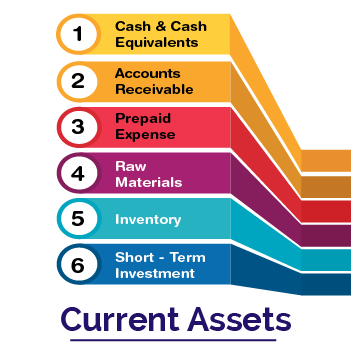# What are Current Assets?

## Current Assets Definition:

A current asset is an asset that a company holds and can be easily sold or consumed and further lead to the conversion of liquid cash. For a company, a current asset is an important factor as it gives them a space to use the money on a day-to-day basis and clear the current business expenses. In other words, the meaning of current assets can be explained as an asset that is expected to last only for a year or less is considered as current assets.## Types of Current Assets:

• Cash and cash equivalent
• Inventory
• Ongoing projects
• Pre-paid expenses
• Account receivable
• Marketable securities

The above mentioned are the obvious list of current assets that are taken into consideration to check the operation cycle of a company within one year.

Also Read: What is the difference between Fixed Assets and Current Assets?

## Key Elements of Current Assets:

Following items are regarded as the key elements of Current Assets

2. Inventories
3. Cash and cash equivalents
4. Short-term investments

## Current Assets Formula

For a company, the current asset in the balance sheet can be calculated as follows.

Current assets=Cash+Cash Equivalents+Inventory+Accounts Receivable+Market Securities+Prepaid Expenses+Other Liquid Assets

## Uses of Current Assets:

• Current Assets can be used as clear regular payments and bills.
• It gives an insight into the company’s cash and liquid position
• Investors and Creditors analyse the company’s current assets closely to understand the risk or benefits involved in the operation.

### Examples of Current Assets

• Cash and equivalents
• Short-term investments (marketable securities)
• Accounts receivable
• Inventory
• Prepaid expenses
• Any other liquid assets

## Total Current Assets

Total current asset is the aggregate of all cash, prepaid expenses, receivables, and inventory on the company’s balance sheet.

Some other formulas that are based on total current assets formula are represented below:

1. Current Ratio = Current Assets ÷ Current Liabilities
2. Quick Ratio = (Current Assets – Inventory + Prepaid Expenses) ÷ Current Liabilities
3. Net Working Capital = Current Assets – Current Liabilities
4. Average Current Assets = (Aggregate Assets for Current Year + Aggregate Assets for Preceding Year) ÷ 2

The concept of ‘Current Assets’ is explained in detail in this article, which is very important for the Commerce students. To learn more such interesting concepts, stay tuned to BYJU’S.

 Important Topics in Accountancy:

#### 1 Comment

1. Stephen markus